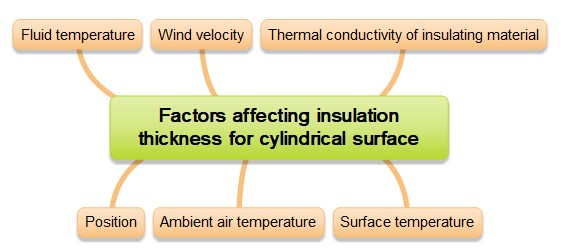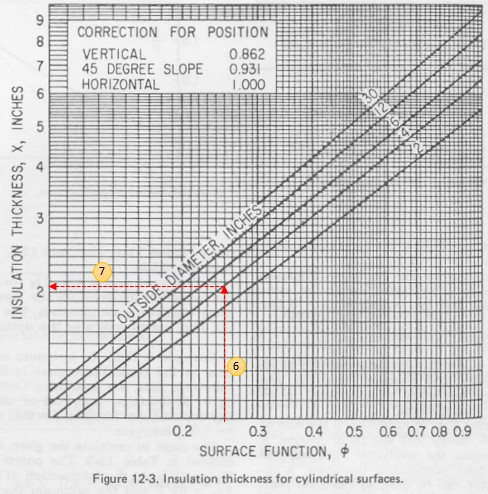Skip to main content# How To Estimate Insulation Thickness

In this post I want to share how to estimate insulation thickness. Charts in this post show how to estimate insulation thickness for stagnant and turbulent air conditions. Be noted, that the charts are based on neglecting the resistances caused by both the metal and the inside film at the inner wall.

Estimating Insulation Thickness for Flat Surface

Insulation thickness for flat surface is a function of:

• Wind velocity
• Ambient air temperature
• Surface temperature
• Fluid temperature
• Thermal conductivity of insulation materialFactors-affecting-insulation-thickness-for-flat-surface

Chart below is used get a parameter called surface function. Surface function value is not a function of shape and orientation of the insulated object. Surface function will later be used to estimate insulation thickness.Estimating-surface-function

To get surface function, we need data below:

• Thermal conductivity of insulation material
• Difference between fluid temperature and surface temperature (∆ti)
• Difference between surface temperature and ambient air temperature (∆to)
• Wind velocity

After we get surface function, then we use chart below to get insulation thickness.Insulation-thickness-for-flat-surfaces

Estimating Insulation Thickness for Cylindrical Surface

Insulation thickness for cylindrical surface is a function of several factors as mentioned above, plus position.Factors affecting insulation thickness for cylindrical surface

The same method is used to estimate insulation thickness for cylindrical surface. First, we need to get surface function using the method mentioned above. Then, we use chart below to get insulation thickness for cylindrical surface. The thickness is a function of outside diameter of object and its position (vertical, horizontal, 45 degree slope).Insulation-thickness-for-cylindrical-surfaces

Example 1: Estimating Insulation Thickness for Flat Surface

Let say we have vertical wall furnace with the following data.

Air temperature               : 85 F

Surface temperature      : 150 F

Fluid temperature           : 650 F

Thermal conductivity of insulation material          : 0.05 Btu/hr.ft.F (at 400 F)

Estimate the insulation thickness.

First, we need to find surface function.

Using above data, we get ∆ti = 500 F and ∆to = 150 F.

Use chart to estimate surface function (I also added step numbers to make it easy to follow)1. Draw a horizontal line from K = 0.05 to ∆ti = 500
2. Drop vertically to ∆ti = 65
3. Draw the horizonal line to left axis
4. Extend a line parallel to the 45-degree slope reference line terminating at the wind velocity base line
5. Draw a horizontal line from the terminating point to the surface function line

By follow the above steps, we get surface function φ = 0.26 for still air (stagnant air).

For a wind velocity 5 ft/s, follow the following steps to get surface function.

4a.   Draw a horizontal line from wind velocity of 5 ft/s to ∆to = 65

5a.   Lift vertically to parallel line as got in step no. 4.

6a.   Draw a horizontal line to the surface function line

We get surface function 0.167 for wind velocity of 5 ft/s.

Second, estimate insulation thickness by using the following chart as a function of the surface position.If the air is still, the thickness on the vertical walls is 2.5 inches.

Example 2: Estimating Insulation Thickness for Cylindrical Surface

Let say we have vertical pipe with the following data.

Outside diameter             : 4 in

Air temperature               : 85 F

Surface temperature      : 150 F

Fluid temperature           : 650 F

Thermal conductivity of insulation material          : 0.05 Btu/hr.ft.F (at 400 F)

Estimate the insulation thickness.

First, we need to find surface function.

Because the condition is the same (thermal conductivity, air temperature, surface temperature, and fluid temperature), then surface function will be the same as previous example, which is 0.26.

Second, estimate insulation thickness by using the following chart as a function of the surface position and multiply by correction factor for positionIf the air is still, the thickness is 2.05 inches x 0.862 = 1.77 inches.

References:

Evans, F. L., Equipment Design Handbook for Refineries and Chemical Plants, Vol. 2, 2nd Ed., Gulf Publishing Co., 1979.